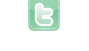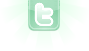# Error

Every time I open Greenfoot, and it defaults to the game i'm currently working on, i get spammed by the following error:
```Exception in thread "AWT-EventQueue-0" java.lang.OutOfMemoryError: Java heap space
```
I dont know what it is, but looks like i'm out of memory. I think its because the code is creating 16k objects, is there a way to get around this error other than shortening my object count?
I "fixed" it by just thinning out the spawns. Still would like to know how to fix this though.
```            while (random_terrain > 0)
{
int q = Greenfoot.getRandomNumber(50);
int w = Greenfoot.getRandomNumber(50);
random_terrain--;
}
random_terrain = Greenfoot.getRandomNumber(500);
while (random_terrain > 0)
{
int q = Greenfoot.getRandomNumber(50);
int w = Greenfoot.getRandomNumber(50);
random_terrain--;
}
random_terrain = Greenfoot.getRandomNumber(500);
while (random_terrain > 0)
{
int q = Greenfoot.getRandomNumber(50);
int w = Greenfoot.getRandomNumber(50);
random_terrain--;
}
random_terrain = Greenfoot.getRandomNumber(500);
while (random_terrain > 0)
{
int q = Greenfoot.getRandomNumber(50);
int w = Greenfoot.getRandomNumber(50);
random_terrain--;
}

while (random_terrain > 0)
{
int q = Greenfoot.getRandomNumber(50);
int w = Greenfoot.getRandomNumber(50);
random_terrain--;
}
random_terrain = Greenfoot.getRandomNumber(500);
while (random_terrain > 0)
{
int q = Greenfoot.getRandomNumber(50);
int w = Greenfoot.getRandomNumber(50);
random_terrain--;
}
random_terrain = Greenfoot.getRandomNumber(500);
while (random_terrain > 0)
{
int q = Greenfoot.getRandomNumber(50);
int w = Greenfoot.getRandomNumber(50);
random_terrain--;
}
random_terrain = Greenfoot.getRandomNumber(500);
while (random_terrain > 0)
{
int q = Greenfoot.getRandomNumber(50);
int w = Greenfoot.getRandomNumber(50);
random_terrain--;
}

while (random_terrain > 0)
{
int q = Greenfoot.getRandomNumber(50);
int w = Greenfoot.getRandomNumber(50);
random_terrain--;
}
random_terrain = Greenfoot.getRandomNumber(500);
while (random_terrain > 0)
{
int q = Greenfoot.getRandomNumber(50);
int w = Greenfoot.getRandomNumber(50);
random_terrain--;
}
random_terrain = Greenfoot.getRandomNumber(500);
while (random_terrain > 0)
{
int q = Greenfoot.getRandomNumber(50);
int w = Greenfoot.getRandomNumber(50);
random_terrain--;
}
random_terrain = Greenfoot.getRandomNumber(500);
while (random_terrain > 0)
{
int q = Greenfoot.getRandomNumber(50);
int w = Greenfoot.getRandomNumber(50);